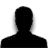Tuts 4 You

# De obfuscate a call?## Recommended PostsI was wondering if anyone could help me with deobfuscating calls removing useless stuff.

I am getting confused with conditional jumps.

I currently have my program step through the call line by line but when it gets to a conditional jump I am not sure what to do. I have tried recursion but that ends in messing up on loops.It depends on the obfuscation method itself ...

Can't give a generic methodIt depends on the obfuscation method itself ...

Can't give a generic methodDotfuscator (.net).

it turns most ifs into switches. I got down resolving the switches but the conditional jumps have me stuck.Oh i thought you mean native code not .NET ...Well what I am stuck at is in both native and cil.Well I have tried many variations of this

`		Instruction[] TraceUntilRet(MethodDefinition m, Instruction cur)		{			List<Instruction> ret = new List<Instruction>();			while (cur != null)			{				if (cur.OpCode == OpCodes.Endfinally || cur.OpCode == OpCodes.Endfilter)				{					cur = cur.Next;					continue;				}				else if (cur.OpCode == OpCodes.Leave)				{					cur.OpCode = OpCodes.Br;				}				else if (cur.OpCode == OpCodes.Leave_S)				{					cur.OpCode = OpCodes.Br_S;				}				else if (cur.Operand is Instruction)				{					Instruction br = (Instruction)cur.Operand;					if (br.OpCode == OpCodes.Ldloc && br.Next.OpCode == OpCodes.Switch)					{						if (cur.Previous != null)						{							if (cur.Previous.Previous != null)							{								ret.RemoveAt(ret.Count - 1);								ret.RemoveAt(ret.Count - 1);								int num = (int)cur.Previous.Previous.Operand;								cur = ((Instruction[])br.Next.Operand)[num];								continue;							}						}					}					if (cur.OpCode == OpCodes.Br || cur.OpCode == OpCodes.Br_S)					{						cur = br;						continue;					}					Instruction[] IF = TraceIntoIf(m, cur, br.Offset);					for (int x = 0; x < IF.Length; x++)					{						ret.Add(IF[x]);					}					if (IF.Length > 0)						ret.Add(cur);					cur = br;					continue;				}				else if (cur.OpCode == OpCodes.Ret)				{					ret.Add(cur);					break;				}				ret.Add(cur);				cur = cur.Next;			}			return ret.ToArray();		}		List<int> Trace = new List<int>();		Instruction[] TraceIntoIf(MethodDefinition m, Instruction cur, int dest) //infi loop (need to have it detect while (something.True())		{			List<Instruction> ret = new List<Instruction>();			Instruction ori = cur;			cur = cur.Next;			while (cur.Offset != dest)			{				if (cur.OpCode == OpCodes.Endfinally || cur.OpCode == OpCodes.Endfilter)				{					cur = cur.Next;					continue;				}				else if (cur.OpCode == OpCodes.Leave)				{					cur.OpCode = OpCodes.Br;				}				else if (cur.OpCode == OpCodes.Leave_S)				{					cur.OpCode = OpCodes.Br_S;				}				else if (cur.Operand is Instruction)				{					Instruction br = (Instruction)cur.Operand;					if (br.OpCode == OpCodes.Ldloc && br.Next.OpCode == OpCodes.Switch)					{						if (cur.Previous != null)						{							if (cur.Previous.Previous != null)							{								ret.RemoveAt(ret.Count - 1);								ret.RemoveAt(ret.Count - 1);								int num = (int)cur.Previous.Previous.Operand;								cur = ((Instruction[])br.Next.Operand)[num];								continue;							}						}					}					if (cur.OpCode == OpCodes.Br || cur.OpCode == OpCodes.Br_S)					{						cur = br;						continue;					}					Instruction[] IF = TraceIntoIf(m, cur, br.Offset);					for (int x = 0; x < IF.Length; x++)					{						ret.Add(IF[x]);					}					if (IF.Length > 0)						ret.Add(cur);					cur = br;					continue;				}				else if (cur.OpCode == OpCodes.Ret)				{					//ret.Add(cur);					break;				}				ret.Add(cur);				cur = cur.Next;			}			return ret.ToArray();		}`
Edited by high6